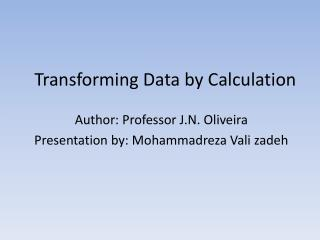DownloadDownload PresentationTransforming Data by Calculation

# Transforming Data by Calculation

Télécharger la présentation## Transforming Data by Calculation

- - - - - - - - - - - - - - - - - - - - - - - - - - - E N D - - - - - - - - - - - - - - - - - - - - - - - - - - -
##### Presentation Transcript

1. Transforming Data by Calculation Author: Professor J.N. Oliveira Presentation by: MohammadrezaValizadeh

2. outline • Why data transformation? • Introducing the Point-free Transform • Data Structures transformation • Data Impedance Mismatch • conclusion

3. Why data transformation? • Watch a calculator

4. • square root Inside a machine these processes happen very much

5. • Such processes happen very much in software systems too. • Each layers stay in different programming paradigms (object-oriented database, relational database)

6. • Data transformation: Middle-ware code to bridge the gap between two different technology layers • Main motivation for data calculation is the need for data mapping

7. • Different data models can be compared via abstraction and representation • Three kinds of fault solution • Loss of data • Confusion among data Data representation • Wrong computation Data processing Helping in preventing any of these from happening in software designs is the main aim of this paper

8. Introducing the Point-free Transform • Converting predicate logic formula into binary relations by removing variables and quantifier(Algebra of programming) • The main principle of the PF-transform: “everything is a binary relation” A R B

9. Data Structures transformation • Data structure: Varity notation , programming language and paradigm

10. • Mapping scenario (transformation) • Type-level mapping of a source data model to a target data model • Two maps (map forward & map backward) between source & target data

11. • The transcription level mapping of source operation to target operation

12. • Sample: Datatype In haskell: In c: